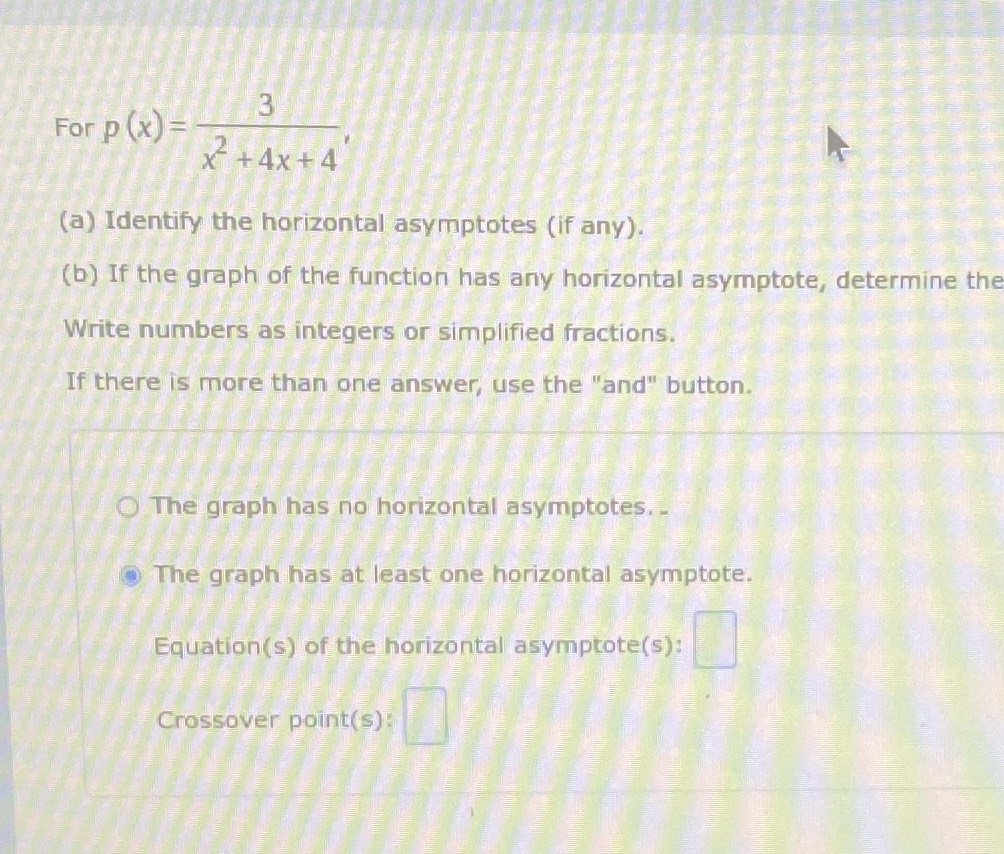### Still have math questions?

Algebra
QuestionFor $$p ( x ) = \frac { 3 } { x ^ { 2 } + 4 x + 4 }$$

(a) Identify the horizontal asymptotes (if any).

(b) If the graph of the function has any horizontal asymptote, determine the Write numbers as integers or simplified fractions.

If there is more than one answer, use the "and" button. .

The graph has no horizontal asymptotes.

The graph has at least one horizontal asymptote.

Equation(s) of the horizontal asymptote(s):

Crossover point(s):

Horizontal Asymptotes: $$y= 0$$